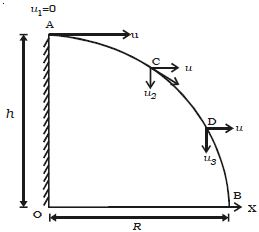Physics

# Explain Motion of Projectile thrown Horizontally

Let consider an object thrown horizontally with a velocity u from a point A. which is at a height h from the horizontal plane OX (Figure). The object acquires the following motions simultaneously:

(i) Uniform velocity with which it is projected in the horizontal direction OX

(ii) Vertical velocity, which is non-uniform due to acceleration due to gravity.Fig: Projectile projected horizontally from the top of a tower

The two velocities are independent of each other. The horizontal velocity of the object shall remain constant as no acceleration is acting in the horizontal direction. The velocity in the vertical direction shall go on changing because of acceleration due to gravity.